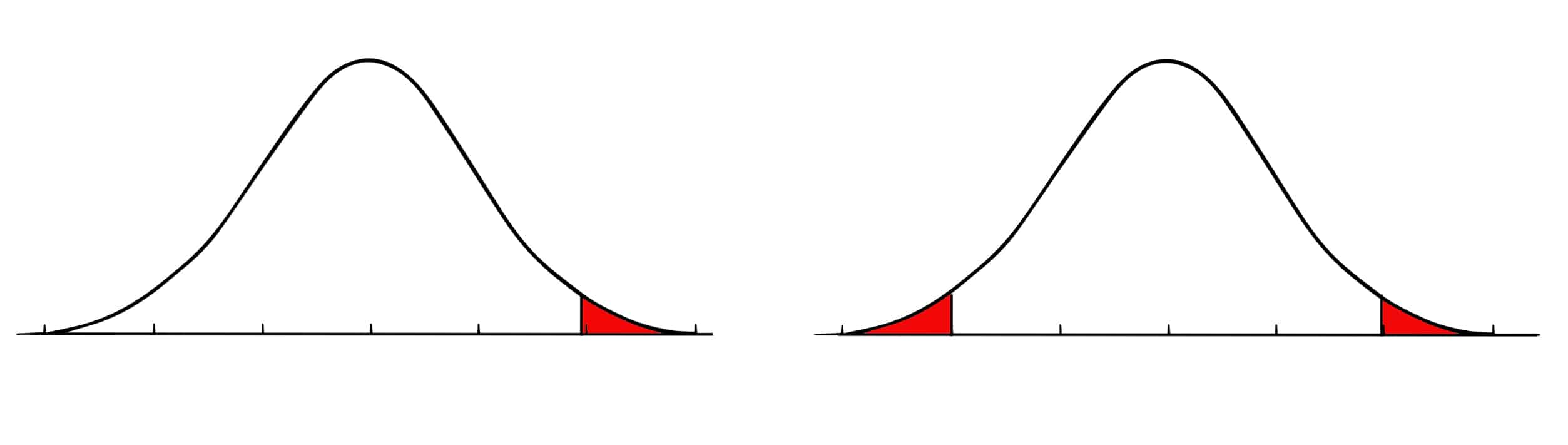# The tails of p

Tabla de contenidos

# Bilateral vs unilateral testingForgive me my friends from the other side of the Atlantic, but I am not thinking about the kind of tails that many perverse minds are. Far from it, today we’re going to talk about a lot more boring tails but that are very important if we want to do a hypothesis testing. And, as usual, we will illustrate the point with an example to try to understand it much better.

Let’s suppose we take a coin and, armed with infinite patience, toss it 1000 times, getting heads 560 times. We all know that the probability of getting heads is 0.5, so if you throw the coin 1000 times we expected to get an average number of 500 heads. But we’ve got 560, so we can consider two possibilities that come to mind immediately.

First, the coin if fair and we’ve got 60 more heads just by chance. This will be our null hypothesis, which says that the probability of getting heads [P(heads)] is equal to 0.5. Second, our coin is not fair, but it is loaded to obtain more heads. This will be our alternative hypothesis (Ha), which states that P(heads) > 0.5.

Well, let’s make a hypothesis testing using one of the binomial probability calculators that are available on the Internet. Assuming the null hypothesis that the coin is fair, the probability to obtain 560 heads or more is 0.008%. Being it lower than 5%, we reject our null hypothesis: the coin is loaded.

## Bilateral vs unilateral testing

Now, if you look well at it, the alternative hypothesis has a directionality towards P(heads) > 0.5, but we could have hypothesized that the coin were not fair without presupposing it was load in favor of heads or tails: P(heads) not equal to 0.5. In this case we would calculate the probability to get a number of heads that were 60 above or below 500, in both directions.

This probability values 0.016%, so we’d reject our null hypothesis and would conclude that the coin is not fair. The problem is that the test doesn’t tell us in what direction it’s loaded but, in the face of the results, we assume it favors heads. In the first example we did a one-tailed test, while in the second we have made a two-tailed test.In the figure, you can see the probability areas in both tests. In the one-tailed test, the red small area on the right represents the probability that the difference from the expected value is due to chance. In the two-tailed test, this area is doubled and located on both sides of the probability distribution. Notice that two-tailed p’s value doubles the one-tailed value.

In our example, both p values are so low that we can reject the null hypothesis in any case. But this is not always so, and there may be occasions when the researcher chooses to do a one-tailed test to get statistical significance that is not possible with the two-tailed test.

And I’m saying one of the two tails because we have calculated the right tail probability, but we could have calculated the probability of the left tail. Consider the unlikely event that even though the coin is loaded favoring tails, we have got more heads just by chance. Our Ha now says that P(heads) < 0.5. In this case we’d calculate the probability that, under the null hypothesis that the coin is fair, we can get 560 tails or less. This p-value is 99.9%, so we cannot reject our null hypothesis that the coin is fair.

## Better one or two tails?

But, what is going on here?, you’ll ask. The first hypothesis test we did allowed us to reject the null hypothesis and the last test says otherwise. Being the same coin and the same data, shouldn’t we have reached the same conclusion?. As it turns out, it seems not. Remember that the fact that we cannot reject the null hypothesis is not the same as to conclude that it is true, a fact we can never be sure of. In the last example, the null hypothesis that the coin is fair is a better option than the alternative that it is loaded favoring tails. However, that does not mean we can conclude that the coin is fair.

## We’re leaving…

You see therefore how important it is to be clear about the meaning of the null and alternative hypothesis when doing a hypothesis testing. And always remember that, even though we cannot reject the null hypothesis it doesn’t mandatorily imply it is true. It could just happen that we haven’t enough power to reject it. This leads me to think about type I and type II errors and their relation with power and sample size. But that’s another story…

This site uses Akismet to reduce spam. Learn how your comment data is processed.

Esta web utiliza cookies propias y de terceros para su correcto funcionamiento y para fines analíticos. Al hacer clic en el botón Aceptar, aceptas el uso de estas tecnologías y el procesamiento de tus datos para estos propósitos. Antes de aceptar puedes ver Configurar cookies para realizar un consentimiento selectivo.    Más información